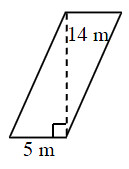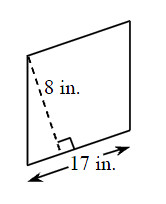### Home > MC2 > Chapter 2 > Lesson 2.3.4 > Problem2-128

2-128.

Find the area of each parallelogram. Show all of your work. Use the Math Notes box if you need help.

See part (a) of Problem 2-116 for help.

1.The area of the parallelogram is equal to the product of its base and its height. Thus, multiply $5(14)$ to find this parallelogram's area.

$5$m ($14$m) $= 70$ m$^2$

1.If you rotate the figure in part (b), you will see that the base is $17$ in. and the height is $8$ in.